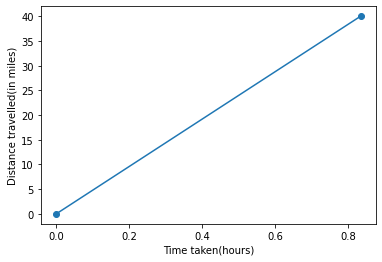1
42
views
14b
Problem

Problem 14b

Chapter 1: Functions and ModelsTextbook ExpertVerified Tutor
23 Oct 2021

Given information

Consider distance traveled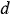(in miles) on the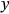axis and time elapsed(in hours) on the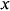axis.

The points given are,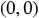and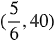.

The equation found in part a) is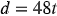(linear model).

Step-by-step explanation

Step 1.

The points are, andThe graph drawn looks like,